direcs  2012-09-30
Circuit Class Reference

Delivers a initialisation for the robot's circuits. More...

`#include <circuit.h>`

Collaboration diagram for Circuit: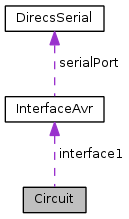[legend]

List of all members.

## Public Slots

bool initCircuit ()
bool initCompass ()
void setRobotState (bool state)
bool sleep ()

## Signals

void compassState (bool state)
void message (QString text)
void robotState (bool state)

## Public Member Functions

Circuit (InterfaceAvr *i, QMutex *m)
bool compassConnected ()
bool isConnected ()
~Circuit ()

## Private Attributes

QString atmelCommand
bool circuitState
QString className
QString commandInitCircuit
QString commandInitCompass
*re#
QString commandSleep
*cc#
bool compassCircuitState
bool firstInitDone
InterfaceAvrinterface1
QMutex * mutex
this will contain the name of this class at runtime for debug messages

## Static Private Attributes

static const char divider = 61
This terminates the serial string for the Atmel controller. 35 = #.
static const unsigned char INIT = 64
*sl#
static const unsigned char INITANSWER = 64
static const bool OFF = false
static const bool ON = true
static const char starter = 42
static const char terminator = 35
This starts the serial string for the Atmel controller. 42 = *.

## Detailed Description

Delivers a initialisation for the robot's circuits.

Definition at line 35 of file circuit.h.

## Constructor & Destructor Documentation

 Circuit::Circuit ( InterfaceAvr * i, QMutex * m )

Definition at line 23 of file circuit.cpp.

 Circuit::~Circuit ( )

Definition at line 45 of file circuit.cpp.

## Member Function Documentation

 bool Circuit::compassConnected ( )
Returns:
The state of the compass module which is connected to the robots circuit (Atmel board).

Definition at line 154 of file circuit.cpp.

Here is the call graph for this function: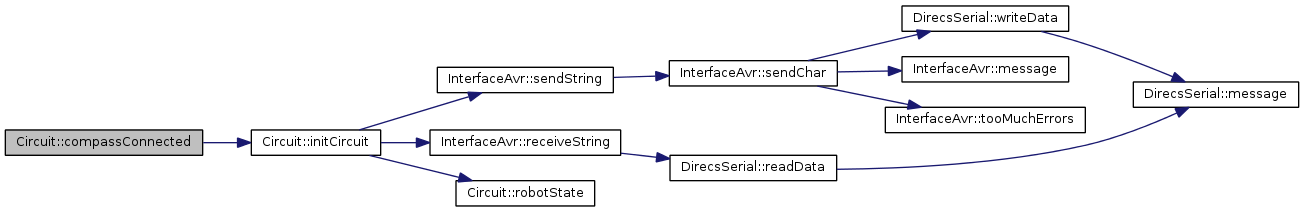void Circuit::compassState ( bool state )
signal

This signal emits the 3D compass module state; it checks if the module is connected to the Atmel board. Not in use, at the moment...

Parameters:
 state can be true or false

Here is the caller graph for this function:bool Circuit::initCircuit ( )
slot

Initialises the robot's circuits. It also checks, if the robot is ON or OFF.

Returns:
true, when the initialization was fine, so the robot is ON.

Definition at line 50 of file circuit.cpp.

Here is the call graph for this function: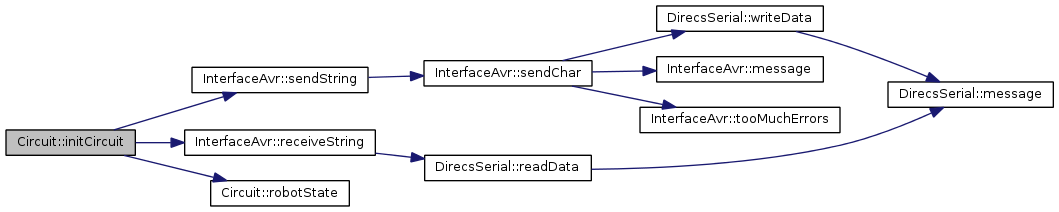Here is the caller graph for this function:bool Circuit::initCompass ( )
slot

Initialises the robot's 3D compass. Actually it checks, if the module is connected or not.

Returns:
true, when connected.

Definition at line 100 of file circuit.cpp.

Here is the call graph for this function: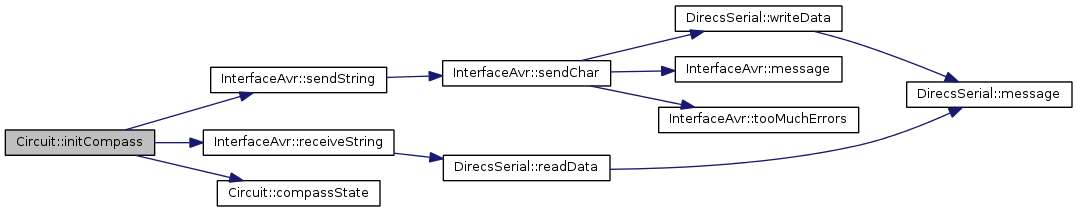bool Circuit::isConnected ( void )
Returns:
The state of the robot (true when connected (and aswers), false when not).

Definition at line 141 of file circuit.cpp.

Here is the call graph for this function: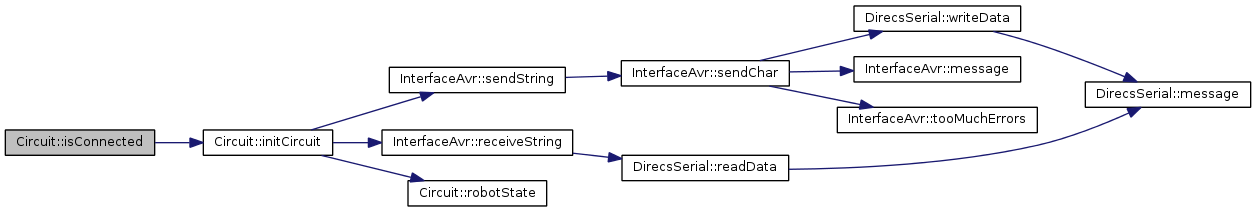void Circuit::message ( QString text )
signal

Sends a string to the GUI log.

Parameters:
 text is the message to be emitted
 void Circuit::robotState ( bool state )
signal

This signal emits the robot (circuit) state to all connected slots, to tell them if the robot is ON or OFF

Parameters:
 state can be true or false

Here is the caller graph for this function: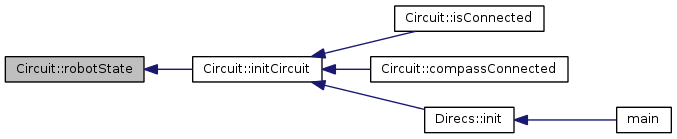void Circuit::setRobotState ( bool state )
slot

This slots takes the robot (circuit) state, to know if the robot is ON or OFF. When the class knows this, unnecessary communication with the interface can be avoided.

Parameters:
 state can be ON or OFF

Definition at line 167 of file circuit.cpp.

 bool Circuit::sleep ( )
slot

Puts the robot's circuits to sleep. This also disables the robot's watchdog!

Returns:
true, when everything was fine

Definition at line 175 of file circuit.cpp.

Here is the call graph for this function: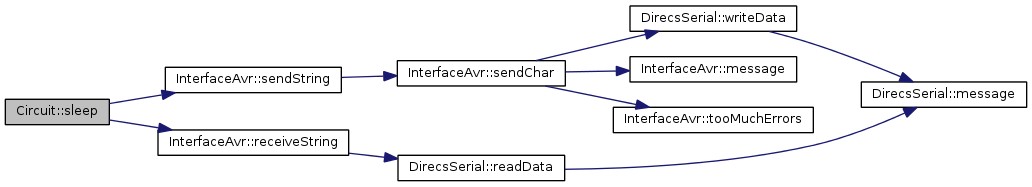Here is the caller graph for this function:## Member Data Documentation

private

Definition at line 110 of file circuit.h.

 QString Circuit::atmelCommand
private

Definition at line 109 of file circuit.h.

 bool Circuit::circuitState
private

Definition at line 119 of file circuit.h.

 QString Circuit::className
private

Definition at line 104 of file circuit.h.

 QString Circuit::commandInitCircuit
private

Definition at line 113 of file circuit.h.

 QString Circuit::commandInitCompass
private

*re#

Definition at line 114 of file circuit.h.

 QString Circuit::commandSleep
private

*cc#

Definition at line 115 of file circuit.h.

 bool Circuit::compassCircuitState
private

Definition at line 121 of file circuit.h.

 const char Circuit::divider = 61
staticprivate

This terminates the serial string for the Atmel controller. 35 = #.

Definition at line 132 of file circuit.h.

private

Definition at line 111 of file circuit.h.

 bool Circuit::firstInitDone
private

Definition at line 120 of file circuit.h.

 const unsigned char Circuit::INIT = 64
staticprivate

*sl#

Definition at line 117 of file circuit.h.

 const unsigned char Circuit::INITANSWER = 64
staticprivate

Definition at line 118 of file circuit.h.

 InterfaceAvr* Circuit::interface1
private

Definition at line 107 of file circuit.h.

 QMutex* Circuit::mutex
mutableprivate

this will contain the name of this class at runtime for debug messages

Definition at line 106 of file circuit.h.

 const bool Circuit::OFF = false
staticprivate

Definition at line 124 of file circuit.h.

 const bool Circuit::ON = true
staticprivate

Definition at line 123 of file circuit.h.

 const char Circuit::starter = 42
staticprivate

example answer string without value from Atmel: *re# example answer string with value from Atmel: *s7=42#

Definition at line 130 of file circuit.h.

 const char Circuit::terminator = 35
staticprivate

This starts the serial string for the Atmel controller. 42 = *.

Definition at line 131 of file circuit.h.

The documentation for this class was generated from the following files: